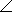# Electrical Engineering - RC Circuits

### Exercise :: RC Circuits - General Questions

21.

A 12 kresistor is in series with a 0.02F capacitor across a 1.2 kHz ac source. If the current is expressed in polar form as I = 0.30° mA, what is the source voltage expressed in polar form?

 A. 45.6 V B. 411.3 V C. 0.411 V D. 4.11 V

Explanation:

No answer description available for this question. Let us discuss.

22.

The complex number 4055° is equivalent to

 A. 55 + j55 B. 40 + j40 C. 45.88 + j65.52 D. 22.94 + j32.76

Explanation:

No answer description available for this question. Let us discuss.

23.

A 120resistor is in parallel with a capacitor with a capacitive reactance of 40. Both components are across a 12 V ac source. What is the magnitude of the total impedance?

 A. 37.9B. 3.7C. 14,400D. 4,800Explanation:

No answer description available for this question. Let us discuss.

24.

A 47resistor and a capacitor with 150of capacitive reactance are in series across an ac source. The impedance, expressed in rectangular form, is

 A. Z = 47+ j150B. Z = 47– j150C. Z = 197D. Z = 103Explanation:

No answer description available for this question. Let us discuss.

25.

A 2 kresistor and a 0.002F capacitor are in series across an ac source. Current in the circuit is 6.50 mA. The true power is

 A. 84.5 mW B. 845 mW C. 13 mW D. 130 mW